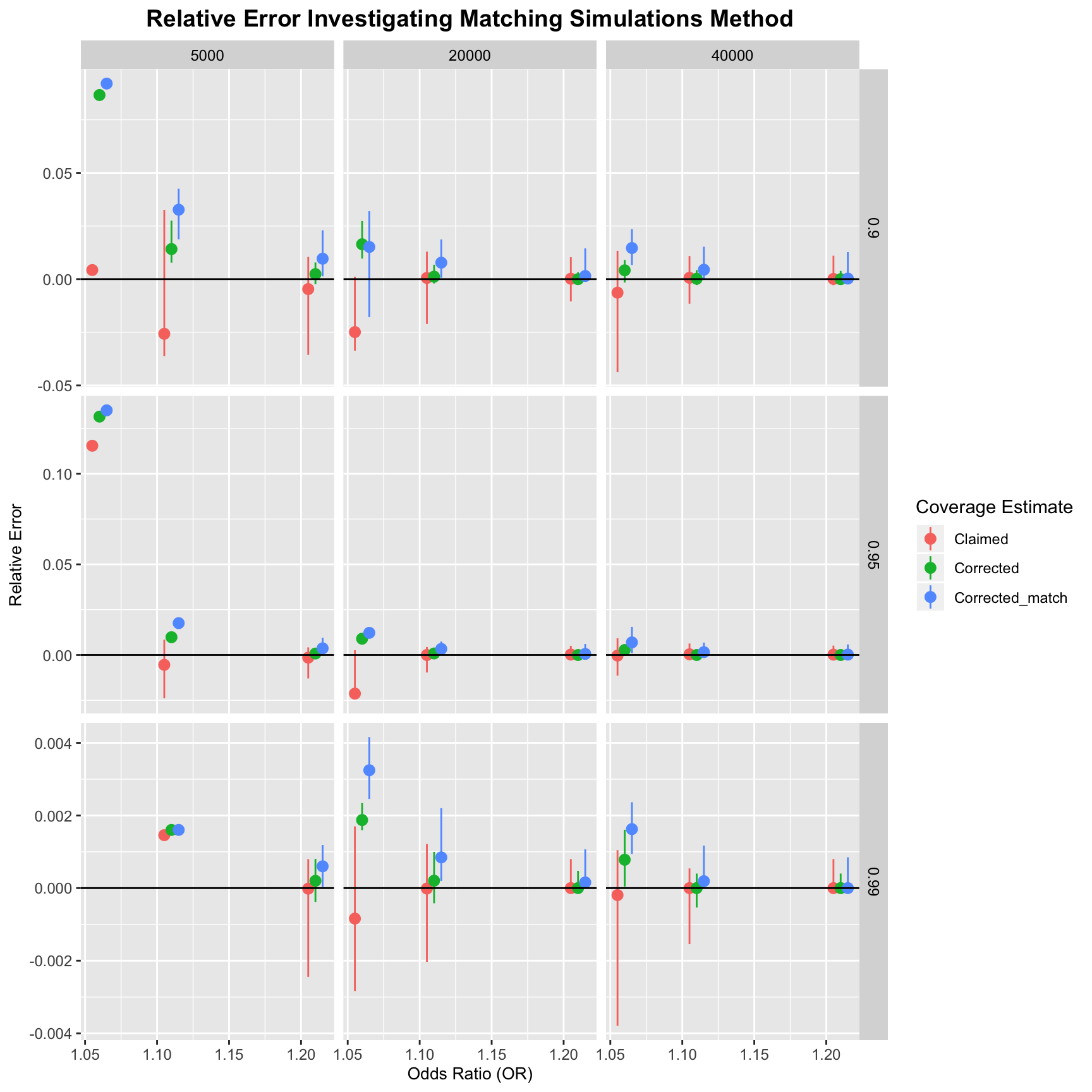## Corrected Coverage Matching Estimate

• This alternative corrected coverage estimate uses only those simulated credible sets where the number of variants matches that of the original credible set to be corrected.

• I am running simulations to implement this method on credible sets with $$nvar < 4$$ (to ensure we are not conditioning on a particularly implausible event - e.g. exactly 120 variants in the set).

• Our standard corrected coverage estimate uses 1000 simulations for each SNP considered causal. Since the matching estimate cuts out SNPs (and we don’t want our estimate to be based only on a handful of samples) I increase the number of simulations for each SNP considered causal to 10K (although this leads to some considered causal SNPs with 10K simulations and some with a handful).

• To get our final corrected coverage estimate, we need to deal with unbalanced samples. To do this, we take a single average by stacking the ‘covered’ binary indicator for each credible set (where the nvar matches what we want). We then make a pp.vect vector of the corresponding posterior probabilities. The length of both of these vectors is sum(nsims). This means that those simulations which have more data (because they have the correct nvar) will be up weighted.

• The simulations are in /APRIL/trim_sims/It seems that this matching method is not very accurate. Perhaps we could limit its use to high power scenarios (large $$\mu$$, e.g. for PTPN22 $$\mu=20$$).